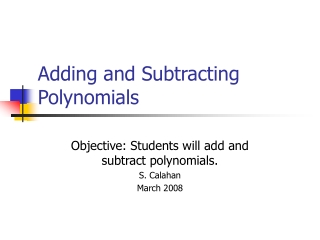DownloadDownload PresentationTélécharger la présentation- - - - - - - - - - - - - - - - - - - - - - - - - - - E N D - - - - - - - - - - - - - - - - - - - - - - - - - - -
##### Presentation Transcript

1. Adding and Subtracting Polynomials Objective: Students will add and subtract polynomials. S. Calahan March 2008

2. Adding • To add polynomials group like terms horizontally or write them in column form, aligning like terms.

3. (3x2 – 4x + 8) + (2x – 7x2 – 5) • Method 1: horizontal • Group like terms. • (3x2 – 7x2) + (-4x + 2x) + (8 – 5) • add like terms. • -4x2– 2x+ 3

4. Method 2: vertical 3x2 – 4x + 8 + -7x2 + 2x -5 - 4x2 – 2x + 3 Notice that terms are in descending orderwith like terms aligned.

5. Subtracting Polynomials • When you subtract polynomials add its opposite or additive inverse. • example:-5x + 3n subtract- (-5x + 3n) Distribute the negative5x – 3n

6. (3n2+13n3+5n) – (7n+4n3) • Method 1: horizontal method (3n2+13n3+5n)–(7n + 4n3) • Distribute the negative (3n2+13n3+5n)+ (-7n– 4n3) • Group like terms. • (13n3–4n3)+3n2+(5n–7n)

7. (13n3 – 4n3) + 3n2 + (5n – 7n) • add like terms • 9n3 + 3n2 – 2n

8. Method 2: Vertical • (3n2+13n3+5n) – (7n+4n3) • Write in descending order • 13n3 + 3n2 + 5n - (4n3 + 7n)

9. 13n3 + 3n2 + 5n- (4n3 + 7n) • Add the opposite • 13n3 + 3n2 + 5n - 4n3 - 7n9n3 + 3n2 – 2n